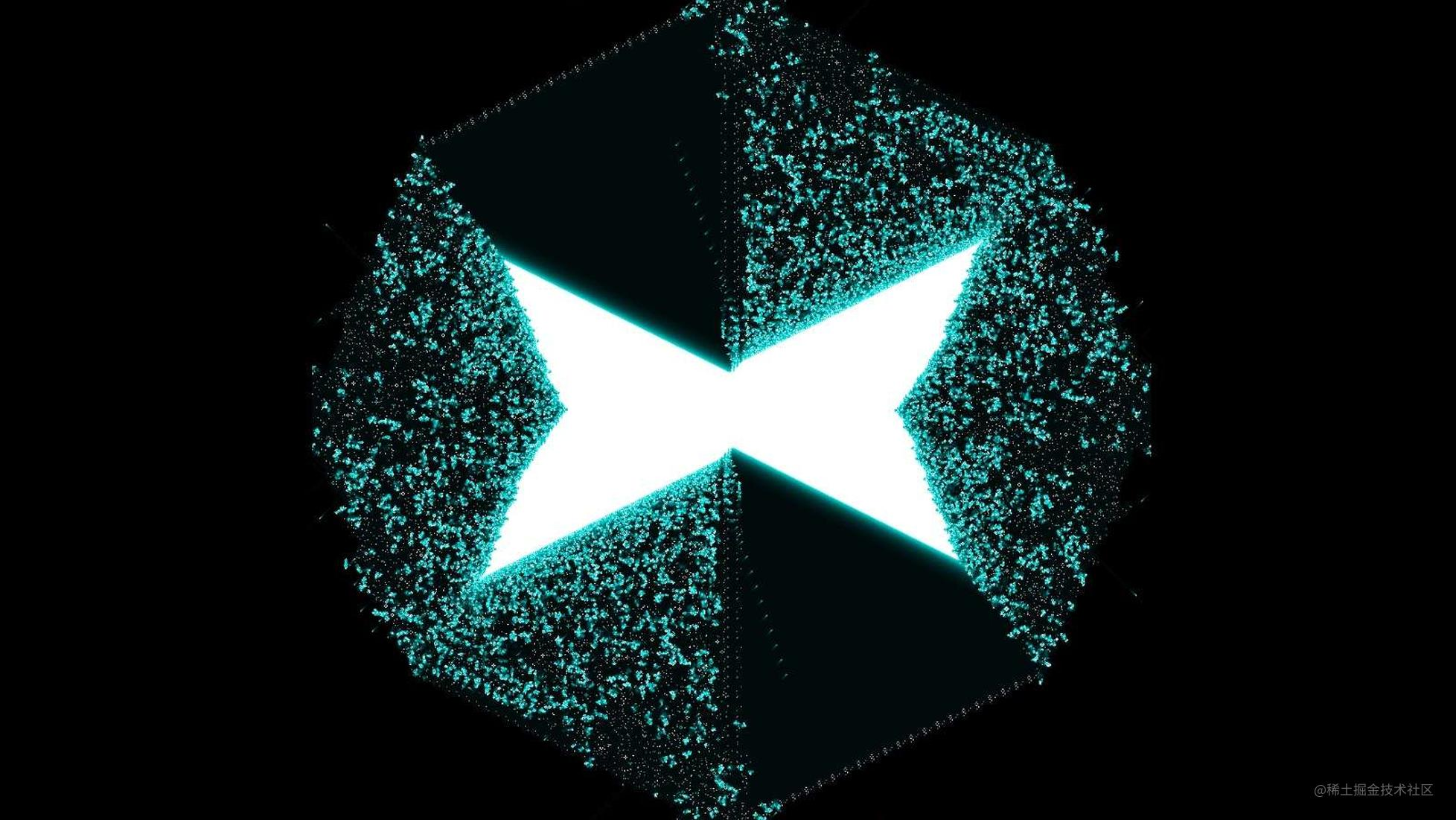# 用网页模拟生命：WebGL 版康威生命游戏

## 背景

• 如果周围活细胞过多，这个细胞会因为资源匮乏而死去（内卷）。
• 如果周围活细胞过少，它也会因为太孤单而死去（人口过少）。
• 如何周围活细胞数量合适，死细胞所在网格也可以变成存活状态（繁殖）。

• 如果当前细胞存活，且周围活细胞数为 2 或 3，保持原样。
• 如果当前细胞存活，且周围活细胞数小于 2，该细胞死亡。
• 如果当前细胞存活，且周围活细胞超过 3 个，该细胞死亡。
• 如果当前细胞死亡，且周围活细胞为 3 个，该细胞转为存活。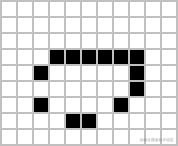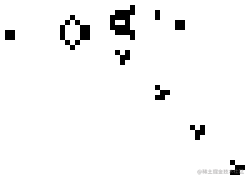## 朴素实现

``````function update (oldCells) {
const newCells = []
for (let i = 0; i < oldCells.length; i++) {
const row = []
for (let j = 0; j < oldCells[i].length; j++) {
const oldCell = get(oldCells, i, j)
const total = (
get(oldCells, i - 1, j - 1) +
get(oldCells, i - 1, j) +
get(oldCells, i - 1, j + 1) +
get(oldCells, i, j - 1) +
get(oldCells, i, j + 1) +
get(oldCells, i + 1, j - 1) +
get(oldCells, i + 1, j) +
get(oldCells, i + 1, j + 1)
)
let newCell = 0
if (oldCell === 0) {
if (total === 3) newCell = 1
}
else if (total === 2 || total === 3) newCell = 1
row.push(newCell)
}
newCells.push(row)
}
return newCells
}

## 加速原理

• 怎样提供 for 循环要遍历的数据？通过提供可按 XY 坐标访问的纹理（Textures）。
• 怎样确定 for 循环遍历的区间范围？通过顶点坐标数组（Buffers）。
• 怎样编写 for 循环里具体的计算逻辑？通过 GLSL 语言写的着色器（Shaders）。

• 提供一个能填满屏幕的矩形（两个三角形），作为渲染的范围。
• 提供一张装有初始状态的位图纹理，作为网格数据。
• 在由 GLSL 语言写的着色器中，对矩形上的每个点（即所谓片元）做周围 8 个点的纹理采样。
• 根据采样结果，在着色器中用简单的 if-else 逻辑做判断，输出颜色。

• 建立两个相同尺寸的纹理，作为渲染目标。
• 初始化时，将 `canvas``img` 中的初始状态上传到第一个纹理。
• 进入 main loop 后，交错地将前一个纹理作为输入，把着色器的计算结果输出到另一个纹理上。

## 基于 Beam 的实现

``````const beam = new Beam(canvas)
const resources = [
// buffers, textures, uniforms...
]

``````// 用于渲染基础图像的着色器

// 构造宽高均为 [-1, 1] 的单位矩形 buffer
const rect = createRect()
const rectBuffers = [
beam.resource(VertexBuffers, rect.vertex),
beam.resource(IndexBuffer, rect.index)
]

// 构造并上传纹理
const textures = beam.resource(Textures)
// 'img' 对应于着色器中的变量名
textures.set('img', { image, flip: true })
// 在 clear 后执行 draw
})

``````const vertexShader = `
attribute vec4 position;
attribute vec2 texCoord;

varying highp vec2 vTexCoord;

void main() {
gl_Position = position;
vTexCoord = texCoord;
}
`

precision highp float;
uniform sampler2D img;

varying highp vec2 vTexCoord;

void main() {
vec4 texColor = texture2D(img, vTexCoord);
gl_FragColor = texColor;
}
`

export const BasicImage = {
buffers: {
position: { type: vec4, n: 3 },
texCoord: { type: vec2 }
},
textures: {
img: { type: tex2D }
}
}

``````void main() {
vec4 texColor = texture2D(img, vTexCoord);
gl_FragColor = texColor;
}

``````function main (img, x, y) {
PIXEL_COLOR = getColor(img, x, y)
}

for (let texCoordX = 0; texCoordX < width; texCoordX++) {
for (let texCoordY = 0; texCoordY < height: texCoordY++) {
main(img, texCoordX, texCoordY)
}
}

``````uniform sampler2D state;
varying vec2 vTexCoord;
const float size = 1.0 / 2048.0; // 像素尺寸换算

void main() {
float total = 0.0;
total += texture2D(state, vTexCoord + vec2(-1.0, -1.0) * size).x > 0.5 ? 1.0 : 0.0;
total += texture2D(state, vTexCoord + vec2(0.0, -1.0) * size).x > 0.5 ? 1.0 : 0.0;
total += texture2D(state, vTexCoord + vec2(1.0, -1.0) * size).x > 0.5 ? 1.0 : 0.0;
total += texture2D(state, vTexCoord + vec2(-1.0, 0.0) * size).x > 0.5 ? 1.0 : 0.0;
total += texture2D(state, vTexCoord + vec2(1.0, 0.0) * size).x > 0.5 ? 1.0 : 0.0;
total += texture2D(state, vTexCoord + vec2(-1.0, 1.0) * size).x > 0.5 ? 1.0 : 0.0;
total += texture2D(state, vTexCoord + vec2(0.0, 1.0) * size).x > 0.5 ? 1.0 : 0.0;
total += texture2D(state, vTexCoord + vec2(1.0, 1.0) * size).x > 0.5 ? 1.0 : 0.0;

vec3 old = texture2D(state, vTexCoord).xyz;
gl_FragColor = vec4(0.0);

if (old.x == 0.0) {
if (total == 3.0) {
gl_FragColor = vec4(1.0);
}
} else if (total == 2.0 || total == 3.0) {
gl_FragColor = vec4(1.0);
}
}

``````// 渲染到屏幕
beam
.clear()

``````// 初始化离屏渲染的 target
const target = beam.resource(OffscreenTarget)

// 将 target 和纹理连接起来
const textures = beam.resource(Textures)
textures.set('img', target)

// 渲染到纹理，已有的渲染逻辑完全不变
beam.clear()
beam.offscreen2D(target, () => {
beam
})

// 在其他着色器中，现在即可使用 textures 下名为 'img' 的纹理

`offscreen2D` 其实是一种可以自定义的 Command。你还可以自行设计出更多「需要清理」的渲染管线操作，将它们封装为 Command，从而借深层嵌套的函数来表达出更复杂的组合，这也是 Beam 的另一个强大之处。

``````const targetA = beam.resource(OffscreenTarget)
const targetB = beam.resource(OffscreenTarget)
let i = 0

const render = () => {
// 交错切换两个 target
const targetFrom = i % 2 === 0 ? targetA : targetB
const targetTo = i % 2 === 0 ? targetB : targetA

beam.clear()
beam.offscreen2D(targetTo, () => {
conwayTexture.set('state', targetFrom)
})

// 将交错渲染的结果，借另一个简单着色器输出到屏幕上
screenTexture.set('img', targetTo)
i++
}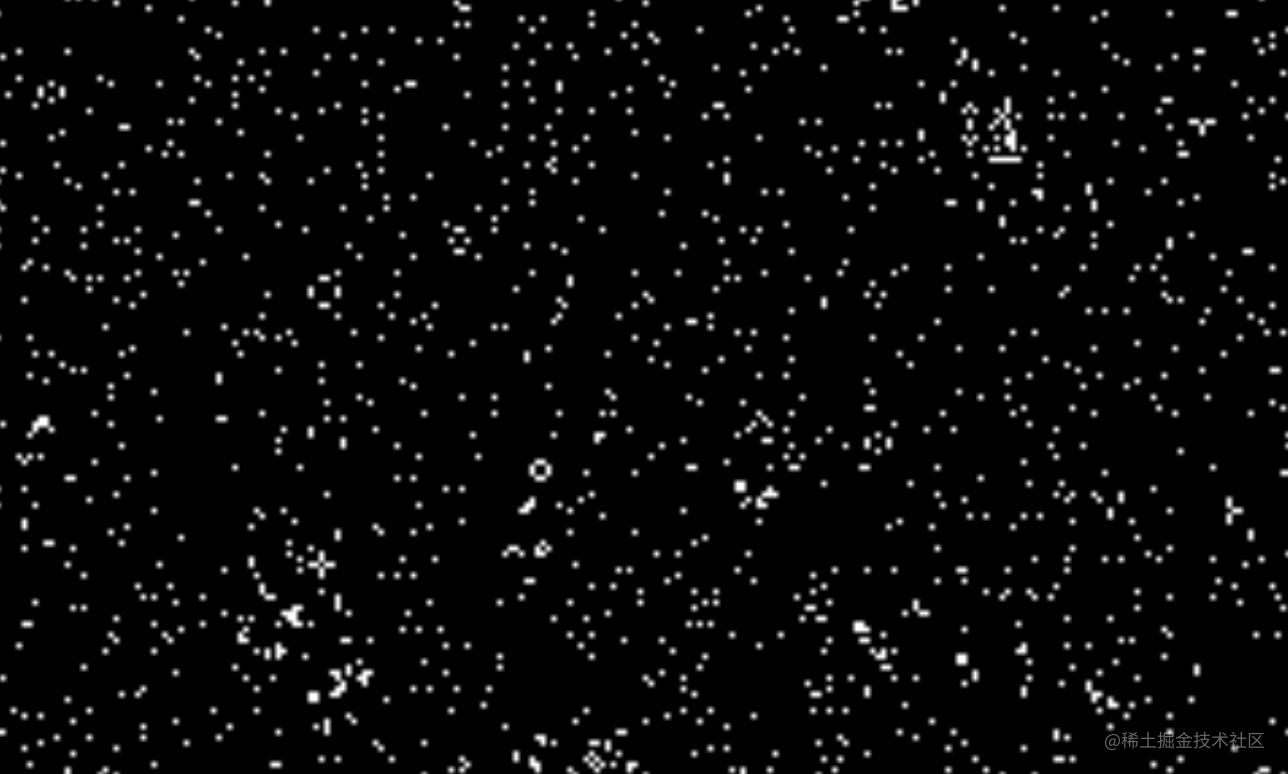``````float decay = 0.95; // 衰减参数

// gl_FragColor = vec4(0.0); // 不再直接置空
gl_FragColor = vec4(0.0, old.yz * decay, 1.0);

// 后续的 if-else...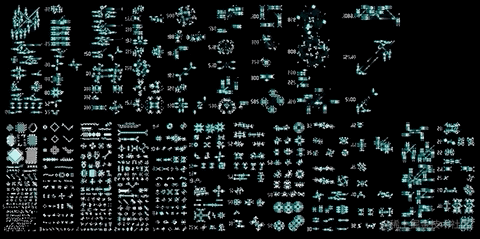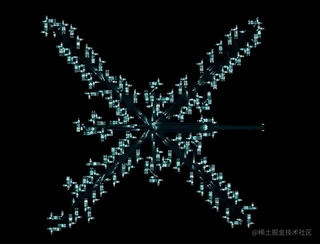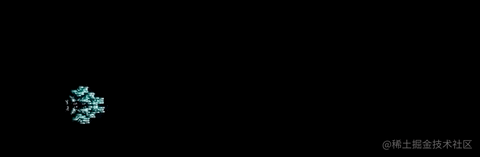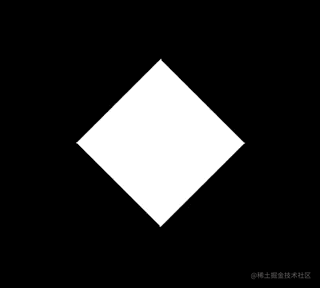## 总结

Beam - Expressive WebGL